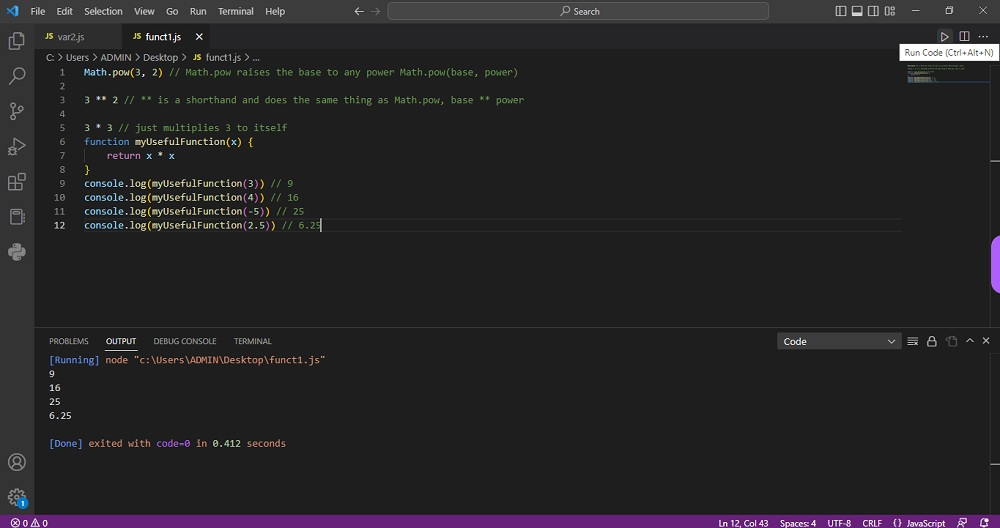# Javascript (Math.pow) Functionby vsasikalabeUpdated: Mar 7, 2023Guide Kit

The Math.pow() function is an in-built function in JavaScript. It uses two parameters - the base and the exponent. It returns a number equal to the base number raised to its exponent. 𝙼𝚊𝚝𝚑.𝚙𝚘𝚠 ( 𝚡 , 𝚢 ) = x y.pow() is used for calculating a number raised to the power of some number.

Syntax of Math.pow()

Math.pow(base, exponent)

Parameters of Math.pow() in JavaScript

It takes two parameters as follows.

• base: It is the base number that needs to be multiplied.
• exponent: It is the value and the number of times a number requires to be multiplied.

In our example, 3**2 does the same operation of Math.pow(). That is base ** power. And for 3 * 3, it multiplies itself by 3.

Here is an example of how to work with Javascript (Math.pow()) Function:Preview of the output that you will get on running this code from your IDE.

### Instructions

Follow the steps carefully to get the output easily.

2. Open the VS Code and install Javascript ES6 Code Snippet and Code Runner from Extensions.
3. Open the new file and save as filename.js
4. Copy the code using the "Copy" button above, and paste it in your javascript file.
5. Run the code using (ctr+alt+N) Run button.

I hope you found this useful. I have added version information in the following sections.

I found this code snippet by searching for ' Javascript Function' in kandi. You can try any such use case!

### Environment Tested

I tested this solution in the following versions. Be mindful of changes when working with other versions.

1. The solution is created in VS Code 1.73.1 version.
2. JavaScript (ES6) code snippets v1.8.0
3. Code Runner v0.11.8

Using this solution, we are able to do Javascript (Math.pow) Function with simple steps. This process also facilities an easy way to use, hassle-free method to create a hands-on working version of code which would help us to do Javascript (Math.pow) Function.

### Support

1. For any support on kandi solution kits, please use the chat
2. For further learning resources, visit the Open Weaver Community learning page